3.2.1 Ideal Gas Laws

Ideal Gas Laws

Boyle’s Law

• Boyle’s Law states:

For a fixed mass of gas at a constant temperature, the pressure p is inversely proportional to the volume V

• This can be expressed in equation form as: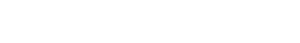• Plotting the pressure against the volume for a gas at a constant temperature on a graph (i.e. pV graph) gives the so-called isothermal curve for the gas

• Plotting the pressure against the reciprocal of the volume (i.e. 1/V) for a gas at constant temperature still gives an isothermal, but this time, the line is straight

• Boyle’s law can be rewritten as follows:

pV = constant

• Which means that:

p1V1 = p2V2

• Where:
• p1 = initial pressure in pascals (Pa) or atmospheres (atm)
• V1 = initial volume in metres cubed (m3) or litres (L)
• p2 = the final pressure in pascals (Pa) or atmospheres (atm)
• V2 = the final volume in metres cubed (m3) or litres (L)

Charles’s Law

• Charle’s Law states:

For a fixed mass of gas at constant pressure, the volume V is directly proportional to the absolute temperature T

• This can be expressed in equation form as: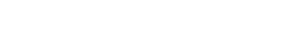• The direct proportionality relationship is only valid if the gas temperature is measured in kelvin (K)
• Plotting the volume against the temperature for a gas at constant pressure gives a straight line along which the gas pressure does not change

• Charles’s law can be rewritten as follows: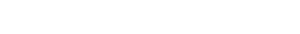• Which means that: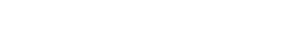• Where:
• V1 = initial volume in metres cubed (m3) or litres (L)
• T1 = initial temperature in kelvin (K)
• V2 = final volume in metres cubed (m3) or litres (L)
• T2 = final temperature in kelvin (K)

Gay Lussac’s Law

• Gay Lussac’s Law states:

For a fixed mass of gas at constant volume, the pressure p is directly proportional to the absolute temperature T

• This can be expressed in equation form as: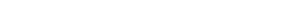• The direct proportionality relationship is only valid if the gas temperature is measured in kelvin (K)
• Plotting the pressure against the temperature for a gas at constant volume gives a straight line along which the gas volume is the same

• Gay Lussac’s law can be rewritten as follows: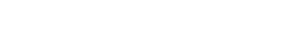• Which means that: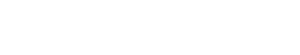• Where:
• p1 = initial pressure in pascals (Pa) or atmospheres (atm)
• T1 = initial temperature in kelvin (K)
• p2 = final pressure in pascals (Pa) or atmospheres (atm)
• T2 = final temperature in kelvin (K)

Gas Laws Combined

• The three gas laws can be combined into one
• For a fixed mass of gas, the following holds: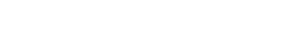• Which means that: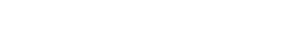• Where:
• p1 = initial pressure in pascals (Pa) or atmospheres (atm)
• V1 = initial volume in metres cubed (m3) or litres (L)
• T1 = initial temperature in kelvin (K)
• p2 = final pressure in pascals (Pa) or atmospheres (atm)
• V2 = final volume in metres cubed (m3) or litres (L)
• T2 = final temperature in kelvin (K)

Worked Example

An ideal gas occupies a volume equal to 5.0 × 10–4 m3. Its pressure is 2.0 × 106 Pa and its temperature is 40°C. The gas is then heated and reaches a temperature of 80°C. It also expands to a new volume of 6.0 × 10–4 m3.

Determine the new pressure of the gas.

Step 1: Write down the given quantities

• Initial volume, V1 = 5.0 × 10–4 m3
• Initial pressure, p1 = 2.0 × 106 Pa
• Initial temperature, T1 = 40°C = 313 K
• Final volume, V2 = 6.0 × 10–4 m3
• Final temperature, T2 = 80°C = 353 K

Remember to:

• Use the appropriate subscripts for initial (i.e. 1) and final (i.e. 2) values
• Convert the temperature from degrees Celsius into Kelvin (K)

Step 2: Write down the equation for the three gas laws combinedStep 3: Rearrange the equation to calculate the unknown final pressure p2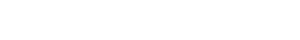Step 4: Substitute numbers into the equationp2 = 1.9 × 106 Pa

Exam Tip

When dealing with gas laws problems, always remember to convert temperatures from degrees Celsius (°C) to kelvin (K).

After you solve a problem using any of the gas laws (or all of them combined), always check whether your final result makes physically sense – e.g. if you are asked to calculate the final pressure of a fixed mass of gas being heated at constant volume, your result must be greater than the initial pressure given in the problem (since Gay- Lussac’s law states that pressure and absolute temperature are directly proportional at constant volume).

CloseClose